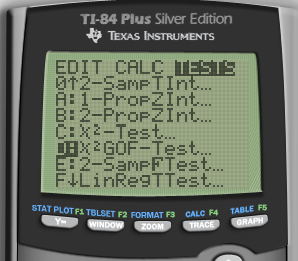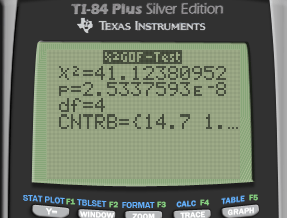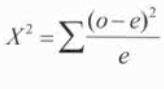# Chi-Squared Goodness of Fit Test

• One categorical variable with counts (or proportion) in each category

• We have seen: products are produced by two machines, machine A produced 15 defective parts in a run of 280, while machine B produced 10 defective parts in a run of 200. Is there a difference in the reliability of these two machines?

• New question type: products are produced by three machines, machine A produced 15 defective parts in a run of 280, while machine B produced 10 defective parts in a run of 200. Is there a difference in the reliability of these machines?

# Practice Question 1

In the past, for a large introductory statistics course, the proportions of students that received grades of A, B, C, D, or F have been, respectively, 0.15, 0.35, 0.30, 0.10, and 0.10

This year, there were 200 students in the class, and following grades were given:

Grade A B C D F
Number 51 79 61 8 1

Test to see whether the distribution of grades this year was different from the distribution in the past?

• Hypothesis

• H0: PA = 0.15, PB = 0.35, PC = 0.30, PD = 0.10, PF = 0.10

• H1: at least one p does not fit the distribution

• Calculate expected values

Grade A B C D F
Observed 51 79 61 8 1
Expected 30 70 60 20 20
• Conditions

• Random

• Independent

• Count: At least 80% of the expected counts are greater than 5 and none are less than 1

• Calculate• Calculate by calculator• P-value

df = k - 1 (k: number of categories)• Interpret

• P < α

• So we reject the null hypothesis and have evidence to support the claim that at least one grade proportion does not fit the expected distribution

# Chi-Squared Test of Homogeneity or Independence/Association

• Two categorical variables

• Homogeneity

• Do two or more sub-groups of a population share the same distribution of a categorical variable (each group has its own sample)

• Do people of different races have the same proportion of smokers to non-smokers.

• Do different education levels have different proportions of Democrats, Republicans, and Independent

• Independence/Association

• Determining whether two categorical variables are associated (variables from a single SRS)

• Is there an association between race and smoking status

• Is there an association between education and voting preference

# Practice Question 2

Girls and boys at an elementary school were sampled and asked about their favorite subject

1. Does favorite subject differ by gender?
Favorite subject Boys Girls Total
Math 96 295 391
English 32 45 77
Social Studies 94 40 134
Total 222 380 602
• Hypothesis

• H0: favorite subject does not differ by gender

• H1: favorite subject does differ by gender

• Expected

• Row Total * Colum Total / Total
• Conditions

• For each sub group, the sample is a SRS

• NO expected cell counts are < 5

• Calculate• Calculate by calculator

![ТЬИ PIus Stver Editj(T ф TEns [NSTRUMENTS гоямдта CALCF4 ](./media/image303.png)![П-84 PIus Stver Editj(n ф TEn.s [NSTRUMENTS гоямдтп CALCF4 п ](./media/image305.png)

• P-value

• df = (r-1)*(c-1)
• Interpret

• P < α

• So we reject the null hypothesis and have evidence to support the claim that favourite subject is different between boys and girls

1. Is favourite subject associated with gender?
• H0: There is no association between favorite subject and gender

• H1: There is an association between favorite subject and gender

# Practice Question 3

You are playing a dice game with a friend. They brought a 6 sided die that you think may not be fair. You conduct an experiment to determine if it is fair. You roll the die 100 times and get following:

Side 1 2 3 4 5 6
Frequency 17 24 15 22 12 10
• Hypothesis

• H0: P1 = P2 = P3 = P4 = P5 = P6

• H1: at least one is not equal

• Expected

• 1/6 = 0.1667
• Conditions

• Random

• Independent

• Expected counts are greater than 5

• Calculate• Calculate by calculator• Interpret

• P > α

• So we fail to reject the null hypothesis and do not have evidence to support the claim that the die is unfair